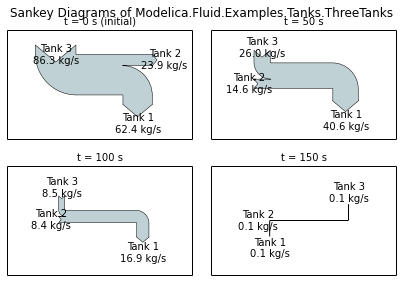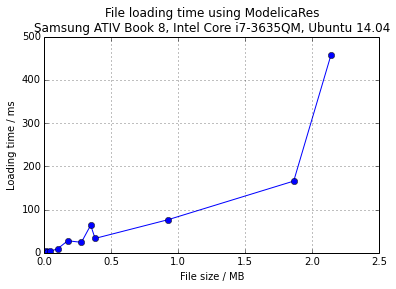This IPython notebook demonstrates some of the advanced features and use cases of ModelicaRes.

First, we'll load the ModelicaRes classes we'll need:

In :
from modelicares import SimRes, SimResList


and some standard modules and settings for this IPython notebook:

In :
import numpy as np
import matplotlib.pyplot as plt
from pandas import DataFrame
%matplotlib inline
%precision 3

Out:
u'%.3f'

## Sankey diagrams¶

SimRes has a built-in method (sankey) to produce Sankey diagrams. We'll plot subfigures with the Sankey diagrams of ThreeTanks at several times over the simulation:

In :
sim = SimRes('ThreeTanks.mat')
sankeys = sim.sankey(title="Sankey Diagrams of Modelica.Fluid.Examples.Tanks.ThreeTanks",
times=[0, 50, 100, 150], n_rows=2, format='%.1f ',
names=['tank1.ports.m_flow', 'tank2.ports.m_flow',
'tank3.ports.m_flow'],
labels=['Tank 1', 'Tank 2', 'Tank 3'],
orientations=[-1, 0, 1],
scale=0.1, margin=6, offset=1.5,
pathlengths=2, trunklength=10)Unfortunately, the formatting arguments (scale, margin, offset, pathlengths, and trunklength) usually require manual adjustment.

## Testing simulations based on criteria¶

As demonstrated in the tutorial, ModelicaRes has a special class for lists of simulation results. We'll use it to load a group of files by wildcard:

In :
sims = SimResList('*', '*/*/*')


Let's see which simulations are in the list:

In :
print(sims)

List of simulation results (SimRes instances) from the following files
in the /media/kld/Storage/Documents/Python/ModelicaRes/examples directory:
ChuaCircuit/2/dsres.mat
ThreeTanks.mat
ChuaCircuit/1/dsres.mat
ChuaCircuit.mat


The directory contained some linearization results, but they were excluded automatically.

Now let's see which variables are available:

In :
sims.names()

Out:
['Time']

Only time is available! names() only returns the variables that the simulations have in common, and there are no others. We'll address that by filtering the list of simulations. To do so, we'll first introduce the concept of a test condition. We'll create a test that checks if a simulation has a variable named "L.L" (indicating that an inductor "L" is at the base of the model):

In :
has_inductor = lambda sim: 'L.L' in sim


Let's see if each simulation passes the test:

In :
[has_inductor(sim) for sim in sims]

Out:
[True, False, True, True]

There's actually another way to access the same information: unique_names(). It returns a dictionary of the variable names that aren't in all of the simulations. The value of each entry is a Boolean list indicating if the variable is in each simulation. For example, we can do:

In :
sims.unique_names()['L.L']

Out:
[True, False, True, True]

Anyway, let's go ahead and filter our simulation list to those that have the inductor:

In :
sims = SimResList(filter(has_inductor, sims))
print(sims)

List of simulation results (SimRes instances) from the following files
in the /media/kld/Storage/Documents/Python/ModelicaRes/examples directory:
ChuaCircuit/2/dsres.mat
ChuaCircuit/1/dsres.mat
ChuaCircuit.mat


The ThreeTanks example has been removed. Now the names method returns so many variables that we'll use a pattern to limit it:

In :
sims.names('L*v')

Out:
[u'L.p.v', u'L.v', u'L.n.v']

In this case, we could have excluded the ThreeTanks example in the first place, but there may be situations where we have a directory of simulation results that we need to sort. We could have also used a test condition that looks at the values of constants, e.g.,

In :
has_small_inductance = lambda sim: 'L.L' in sim and sim['L.L'].value() < 14


This idea isn't limited to Boolean test functions. We could just as easily map a cost function to a list of simulations.

## Indexing lists of simulations¶

As mentioned in the tutorial, the get item method can be used on a SimResList of simulations to retrieve an attribute across all of the simulations, e.g.,

In :
sims['L.L'].value()

Out:
[10.0, 18.0, 18.0]

However, the method is overloaded so that it can still be used to index a simulation from the list of simulations:

In :
sims['L.L'].value()

Out:
10.0

In this case, the first index was for the simulation result (a SimRes instance) and the second was for the variable within the result.

The in operator is also overloaded. It can be used to check if a variable is in all of the simulations:

In :
'L.L' in sims

Out:
True

or if a simulation is in the list of simulations:

In :
sims in sims

Out:
True

However, it is not appropriate to overload some Python list methods in this context. Those that are not overloaded (e.g., insert, remove, pop, index, and count) only accept integer indices or the actual list elements (SimRes instances) as applicable, not variable names.

It is possible to use slices to extract a SimResList with selected simulations:

In :
print(sims[:2])

[SimRes('/media/kld/Storage/Documents/Python/ModelicaRes/examples/ChuaCircuit/2/dsres.mat'), SimRes('/media/kld/Storage/Documents/Python/ModelicaRes/examples/ChuaCircuit/1/dsres.mat')]


Compare this to the last print(sims) in the previous section.

Note that the call method is not available for a list of simulations (only for a single simulation; see __call__). The return value would be too confusing. However, it's possible to retrieve information from multiple variables manually, e.g.,

In :
{"Final value of " + name: sims[name].FV() for name in ['C1.v', 'C2.v', 'L.v']}

Out:
{'Final value of C1.v': [2.2080979, 2.4209836, 2.4209836],
'Final value of C2.v': [0.0014105374, -0.22792035, -0.22792035],
'Final value of L.v': [-0.012604702, -0.25352862, -0.25352862]}

Here we iterated over the variables. For a single simulation we could do this automatically:

In :
sims(['C1.v', 'C2.v', 'L.v']).FV()

Out:
[2.2080979, 0.0014105374, -0.012604702]

where these values form the first "column" of the previous dictionary.

## Speed considerations¶

In the tutorial, we saw two ways to access variables: the get item method (square brackets) and the call method (parentheses). The get item method allows access to only one variable at a time but is a little faster:

In :
sim = SimRes('ChuaCircuit.mat')

In :
timeit sim['L.i'].values()

1000000 loops, best of 3: 1.33 µs per loop

In :
timeit sim('L.i').values()

1000000 loops, best of 3: 1.58 µs per loop


Both of these approaches consist of two steps: accessing the variable (sim['L.i'] or sim('L.i')) and reading the values. The first step takes almost as much time as the second, but it only needs to be performed once. If we need to access a variable or a group of variables multiple times (to get their values, units, times, etc.), it's best to assign a variable to the entry or entries:

In :
Li = sim['L.i']


With the first step out of the way, we can now retrieve information more quickly:

In :
timeit Li.values()

1000000 loops, best of 3: 712 ns per loop


The same holds for the call method. We'll access the voltages of all of the components in the ChuaCircuit:

In :
voltages = sim.names('^[^.]*.v\$', re=True)


Running both steps at once

In :
timeit sim(voltages).values()

100000 loops, best of 3: 9.82 µs per loop


takes almost twice as long as the second step alone:

In :
v = sim(voltages)

In :
timeit v.values()

100000 loops, best of 3: 6.06 µs per loop


As shown in the plot below, the simulation file loading time is not extremely quick, but it is but manageable -- slightly better than half a second for a 2 MB file. The constants_only initialization option of SimRes saves about 15 to 25% of the load time and may be useful if it is only necessary to read parameters.

In :
d = DataFrame({'file size / B':[14679, 19835, 43027, 100403, 176259, 278277,
347461, 380311, 924934,  1863585, 2141881],
'load time / s': [0.0044, 0.0012, 0.00334, 0.0093, 0.0279, 0.0242,
0.0639, 0.0333, 0.0766, 0.166, 0.458]})
plt.plot(d['file size / B']/1e6, d['load time / s']*1e3, 'o-')
"Samsung ATIV Book 8, Intel Core i7-3635QM, Ubuntu 14.04")
plt.xlabel('File size / MB')
plt.grid(True)There appears to be about a 60% memory overhead associated with the data. A 278.3 kB file took approximately 4.5 GB of system memory when loaded 10,000 times:

In :
4.5e9/(1e4*278.3e3) - 1

Out:
0.617

## Contributing¶

Now you've seen the main features of ModelicaRes besides the exps module (tools to help set up and run simulation experiments). If there is a compelling use case or feature you'd like to see added, please consider developing it yourself and sharing it by a pull request to the master branch of the GitHub repository. The ModelicaRes source code is well documented and organized to allow expansion.